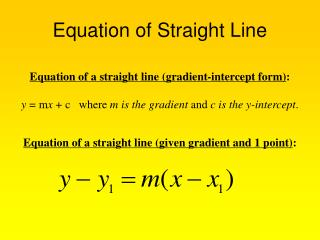DownloadDownload PresentationEquation of Straight Line

# Equation of Straight Line

Télécharger la présentation## Equation of Straight Line

- - - - - - - - - - - - - - - - - - - - - - - - - - - E N D - - - - - - - - - - - - - - - - - - - - - - - - - - -
##### Presentation Transcript

1. Equation of Straight Line Equation of a straight line (gradient-intercept form): y = mx + c where m is the gradient and c is the y-intercept. Equation of a straight line (given gradient and 1 point):

2. Finding equations Find the equation of the straight line joining (2, 4) and (–2, 3) (–2, – 4) and (1, –7)

3. Finding equations Find the equation of the lines, given the gradient and the coordinate of a point lying on the line. (a) m = 3, (1, 1) (b) m = 0.5, (5, 7)

4. Finding Equations • What is the equation of the line which has gradient 2 and which passes through the origin?

5. Finding Equations • Find the equation of the straight line that is parallel to and bisects the line segment joining the points (3, 1) and (1, –5).

6. Finding Equations Find the values of k if the line is parallel to

7. Finding Equations Given the line, find • its gradient; • the coordinates of the point at which it cuts the x-axis.

8. Double-intercept form General form Gradient-intercept form *Given gradient and 1 point Straight line equations of different forms

9. Collinear Points 3 points are collinear if gradient AB = gradient BC